Online Digital Electronics Test - Digital Electronics Test 4Loading Test...

Instruction:

• This is a FREE online test. DO NOT pay money to anyone to attend this test.
• Total number of questions : 20.
• Time alloted : 30 minutes.
• Each question carry 1 mark, no negative marks.
• DO NOT refresh the page.
• All the best :-).

1.

Once a signal is digitized, the information it contains does not ________ as it is processed.

A.
 complainB.
 stiffenC.
 compressD.
 deteriorateCorrect Answer: Option D

Learn more problems on : Digital Concepts

2.

A computer will use ASCII code to store information internally.

A.
 TrueB.
 FalseCorrect Answer: Option B

Learn more problems on : Number Systems and Codes

3.

The logic expression for a NOR gate is ________.

A.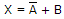B.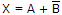C.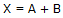D.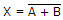Correct Answer: Option D

Learn more problems on : Logic Gates

4.

It is important to memorize logic symbols, Boolean equations, and truth tables for logic gates.

A.
 TrueB.
 FalseCorrect Answer: Option A

Learn more problems on : Logic Gates

5.

The output of an AND gate is HIGH when any input is HIGH.

A.
 TrueB.
 FalseCorrect Answer: Option B

Learn more problems on : Combinational Logic Analysis

6.

For the device shown here, assume the D input is LOW, both S inputs are LOW, and the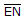input is LOW. What is the status of the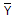outputs?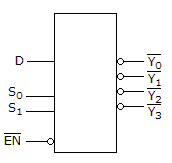A.
 All are HIGH.B.
 All are LOW.C.
 All but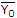are LOW.D.
 All butare HIGH.Correct Answer: Option D

Learn more problems on : Combinational Logic Circuits

7.

Looping on a K-map always results in the elimination of:

A.
 variables within the loop that appear only in their complemented form.B.
 variables that remain unchanged within the loop.C.
 variables within the loop that appear in both complemented and uncomplemented form.D.
 variables within the loop that appear only in their uncomplemented form.Correct Answer: Option C

Learn more problems on : Combinational Logic Circuits

8.

VHDL was created as a very flexible language and it allows us to define the operation of clocked devices in the code without relying on logic primitives.

A.
 TrueB.
 FalseCorrect Answer: Option A

9.

A(n) ________ one-shot starts a pulse in response to a trigger and will restart the internal pulse timer every time a subsequent trigger edge occurs before the pulse is complete.

A.
 non-retriggerableB.
 retriggerableC.
 high-level triggeredD.
 edge-triggeredCorrect Answer: Option B

10.

The condition occurring when two or more devices try to write data to a bus simultaneously is called ________.

A.
 address decodingB.
 bus contentionC.
 bus collisionsD.
 address multiplexingCorrect Answer: Option B

Learn more problems on : Memory and Storage

11.

Select the statement that best describes Read-Only Memory (ROM).

A.
 nonvolatile, used to store information that changes during system operationB.
 nonvolatile, used to store information that does not change during system operationC.
 volatile, used to store information that changes during system operationD.
 volatile, used to store information that does not change during system operationCorrect Answer: Option B

Learn more problems on : Memory and Storage

12.

ROMs retain data when the ________.

A.
 power is offB.
 power is onC.
 system is downD.
 all of the aboveCorrect Answer: Option D

Learn more problems on : Memory and Storage

13.

Address decoding for dynamic memory chip control may also be used for:

A.
 controlling refresh circuitsB.
 read and write controlC.
 chip selection and address locationD.
 memory mappingCorrect Answer: Option C

Learn more problems on : Memory and Storage

14.

ROM access time is defined as ________.

A.
 how long it takes to program the ROM chipB.
 being the difference between the READ and WRITE timesC.
 the time it takes to get valid output data after a valid address is appliedD.
 the time required to activate the address lines after the ENABLE line is at a valid levelCorrect Answer: Option C

Learn more problems on : Memory and Storage

15.

The JTAG signals are named TDI, TDO, TMS, and TCK.

A.
 TrueB.
 FalseCorrect Answer: Option A

Learn more problems on : Programmable Logic Device

16.

Most PAL devices have a tristate buffer driving the input pins.

A.
 TrueB.
 FalseCorrect Answer: Option B

Learn more problems on : Programmable Logic Device

17.

An EPM 7128S in a ________ PQFP package has 12 I/O per LAB plus 4 additional input-only pins for a total of 100 pins.

A.
 100-pinB.
 120-pinC.
 140-pinD.
 160-pinCorrect Answer: Option D

Learn more problems on : Programmable Logic Device

18.

The SUBDESIGN section defines the input and output of the logic circuit block.

A.
 TrueB.
 FalseCorrect Answer: Option A

Learn more problems on : Describing Logic Circuits

19.

One CASE construct inside another CASE construct is called a do-loop.

A.
 TrueB.
 FalseCorrect Answer: Option B

Learn more problems on : Digital System Projects Using HDL

20.

A logic circuit that provides a HIGH output if one input or the other input, but not both, is HIGH, is a(n):

A.
 Ex-NOR gateB.
 OR gateC.
 Ex-OR gateD.
 NAND gateCorrect Answer: Option C

Learn more problems on : Ex-OR and Ex-NOR Gates